# SVD原理及在SLAM中的应用

## Mar 17,2018   4636 words   17 min

Tags: SLAM

#### 一、线性变换与矩阵乘法

$\begin{pmatrix} 3 & 0\\ 0 & 1 \end{pmatrix}\begin{pmatrix} x\\ y \end{pmatrix}=\begin{pmatrix} 3x\\ y \end{pmatrix}$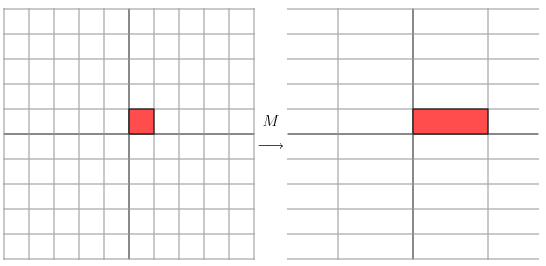$\begin{pmatrix} 2 & 1\\ 1 & 2 \end{pmatrix}$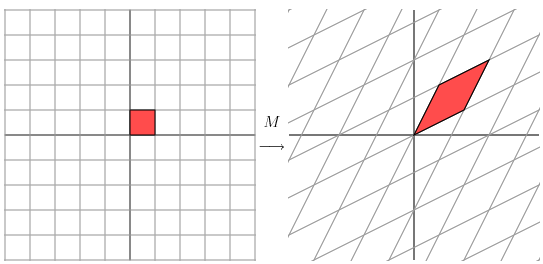$A=\begin{pmatrix} x & y & z \end{pmatrix}=\begin{pmatrix} 1 & 0 & 0\\ 0 & 1 & 0\\ 0 & 0 & 1 \end{pmatrix}$

$AV=\begin{pmatrix} x & y & z \end{pmatrix}\begin{pmatrix} 3\\ 4\\ 5 \end{pmatrix}=3x+4y+5z$

A的列向量是标准基，描绘的是笛卡尔空间。向量V只是一个计量每个轴上有几个单位长度的东西。在笛卡尔空间中，X方向上的1个单位长度可以用矩阵乘法算得表示如下

$IV = \begin{pmatrix} 1 & 0 & 0\\ 0 & 1 & 0\\ 0 & 0 & 1 \end{pmatrix}\begin{pmatrix} 1\\ 0\\ 0 \end{pmatrix}=\begin{pmatrix} 1\\ 0\\ 0 \end{pmatrix}=x$

$A=\begin{pmatrix} 1 & 1 & 1\\ 1 & 2 & 2\\ 1 & 2 & 3 \end{pmatrix}$ $\begin{pmatrix} 1 & 1 & 1\\ 1 & 2 & 2\\ 1 & 2 & 3 \end{pmatrix}\begin{pmatrix} 1\\ 0\\ 0 \end{pmatrix}=\begin{pmatrix} 1\\ 1\\ 1 \end{pmatrix}$

$C=A\cdot B$

$C=A\cdot B \cdot I=A \cdot W$

I为单位阵，它的列向量可以看成是笛卡尔空间的标准基底。W的列向量就表示I经过矩阵B变换以后的基底。而C的列向量就是W空间中的基底经过矩阵A变换后的新空间C的基底。

#### 三、奇异值分解

$M=U\Sigma V^{*}$

$M=\begin{pmatrix} 1 & 1\\ 0 & 1 \end{pmatrix}$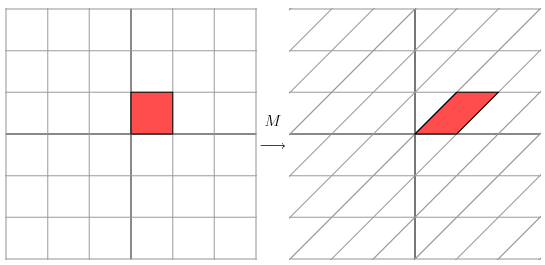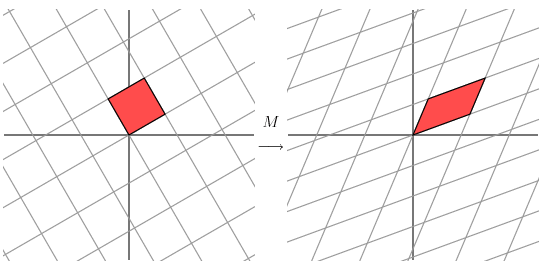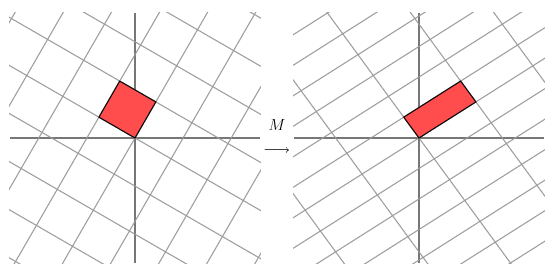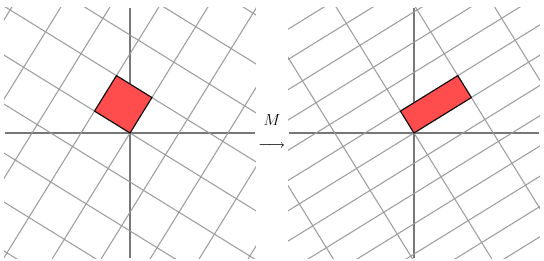$MVI=U\Sigma$

$M=U\Sigma V^{T}$

V的列向量就是$$M^{T}M$$的特征向量，U的列向量就是$$MM^{T}$$的特征向量。Σ的对角线元素(非零奇异值)就是$$M^{T}M$$或$$MM^{T}$$的非零特征值的均方根。#### 四、SVD的应用

##### 1.求伪逆

$M=U\Sigma V^{*}$

$M^{+}=V\Sigma^{+} U^{*}$

$$\Sigma^{+}$$是Σ的伪逆，是将Σ主对角线上每个非零元素都求倒数之后再转置得到的。求伪逆通常可以用来求解最小二乘法问题。

##### 3.解方程

• [V D] = eig(A'A);D为A’A的特征值对角矩阵，V为对应的特征向量。找到最小特征值对应的V中的特征向量即为最小二乘解。

• 使用SVD分解矩阵A，[U S V] = svd(A); U由AA’的特征向量组成，V由A’A的特征向量组成。A’在Matlab中表示A的转置.因此，奇异值矩阵S中最小的奇异值对应的V中的奇异向量即为最小二乘解。又由于S中的奇异值一般都是从大到小排列的，因此一般V的最右一列对应的数字就是方程的解。

#### 六、参考资料

• https://blog.csdn.net/heyijia0327/article/details/26757435
• https://blog.csdn.net/heyijia0327/article/details/26760737
• https://blog.csdn.net/heyijia0327/article/details/26762531
• https://blog.csdn.net/heyijia0327/article/details/26763903
• https://www.ams.org/publicoutreach/feature-column/fcarc-svd
• https://blog.stata.com/2011/03/03/understanding-matrices-intuitively-part-1/